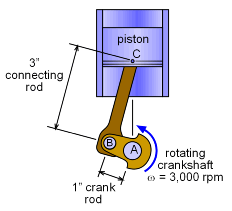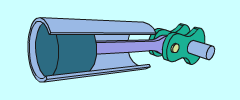Ch 5. Rigid Body General Motion Multimedia Engineering Dynamics Fixed Axis Rotation Plane Motion Velocities Zero Velocity Point Plane Motion Accelerations Multiple Gears Rot. Coord. Velocities Rot. Coord. Acceleration
 Chapter - Particle - 1. General Motion 2. Force & Accel. 3. Energy 4. Momentum - Rigid Body - 5. General Motion 6. Force & Accel. 7. Energy 8. Momentum 9. 3-D Motion 10. Vibrations Appendix Basic Math Units Basic Equations Sections Search eBooks Dynamics Fluids Math Mechanics Statics Thermodynamics Author(s): Kurt Gramoll ©Kurt GramollDYNAMICS - CASE STUDY IntroductionProblem Diagram A car engine manufacturer needs to know the maximum linear velocity of the piston head in a new prototype engine. The technicians know the rotational speed of the crankshaft but are unable to determine the linear velocity of the actual piston head. What is known: The crankshaft rotates at 3,000 rpm. The crank rod length is 1 inch. The connecting rod length is 3 inches. The piston head is constrained to move in the vertical direction. Question What is the maximum velocity of the piston head over a complete rotation?Linear Motion Conversion to Rotation Motion Approach The problem can be solved by splitting it into two parts. First, solve for the velocity of point B, vB, using bar AB, and then solve for the velocity of point C, vC, using vB and bar BC. Apply the relative velocity equation for a rigid body for both bar AB and bar BC, vB = vA + vB/A

Practice Homework and Test problems now available in the 'Eng Dynamics' mobile app
Includes over 400 problems with complete detailed solutions.
Available now at the Google Play Store and Apple App Store.